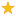quizzes  Forums# Gastronomy

created by coldplay on 24 Apr 08Medium level (60% of success)    10 questions - 533 players
What do we eat in the world ?1
Where is the moussaka from ?

2
Where is the Paella from ?

3
Where is the Osso Buco from ?

4
Where is the mezze from ?

5
Where is the Och from ?

6
Where is the Anticuchos from ?

7
Where is the Tempura from ?

8
Where is the Nasi Lemak ?

9
Where is the Waterzooï from ?

10
Where is the Bouillabaisse from ?

Quiz.biz does not certify the quizzes to be reliable, contact Coldplay !Comment this Quiz
If you want to rank and comment this quizz, login in or create an account !